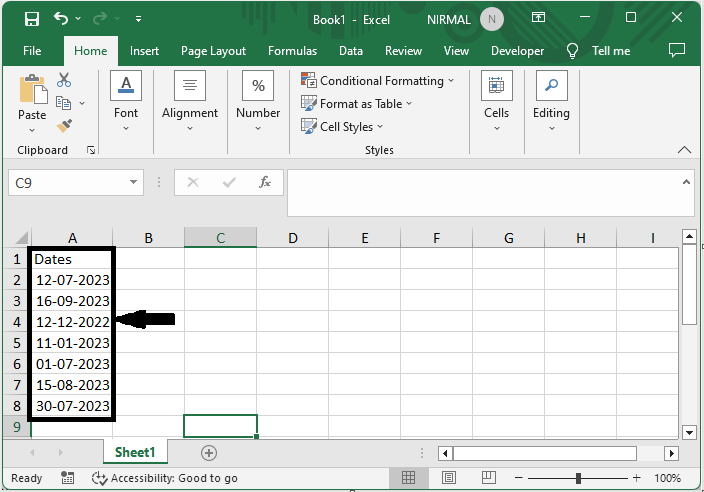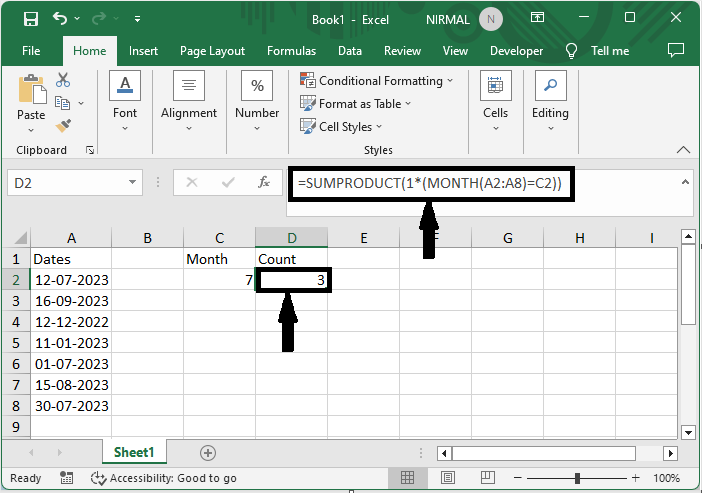# How to Countif by Date/Month/Year and Date Range in Excel?

Excel is a strong tool that provides a variety of formulae and functions to carry out different computations and analyses on your data. COUNTIF, which enables you to count the number of cells in a range that satisfy a particular requirement, is one of the most often used functions. This tutorial will concentrate on using the COUNTIF function to count cells according to date criteria, such as by date, month, year, and within a given period range.

By the time you finish this video, you will have a firm grasp on how to use Excel's COUNTIF function to gain valuable insights from your data, particularly when working with date-based criteria. This article will give you step-by-step directions and examples to help you learn the skill of counting cells by date and date range, whether you're a novice or an intermediate Excel user.

## Countif by Date/Month/Year and Date Range in Excel

Here, we can complete the task directly using the formula. So let us see a simple process to know how you can count by date, month, year, and date range in Excel.

Consider an Excel sheet where you have list of dates as similar to the below image.First, to count by month, click on an empty cell, in this case cell D2, and enter the formula as =SUMPRODUCT(1*(MONTH(C3:C16)=G2)) and click enter to complete the task. In the formula, C3:C16 is the range of cells, and G2 is the month.

Empty cell > Formula > Enter.This is how you can count by date, month, year, and date range in Excel.

Note

• To count by the year, use the formula =SUMPRODUCT(1*(YEAR(C3:C16)=1988)).

• To count by the date, use the formula =COUNTIF(B2:B15, 1992-8-16").

• To count if by the date range, use the formula =COUNTIF(B2:B15, ">" & "1990-1-1").

## Conclusion

In this tutorial, we have used a simple example to demonstrate how you can count by date, month, year, and date range in Excel to highlight a particular set of data.

Updated on: 22-Aug-2023

4K+ Views